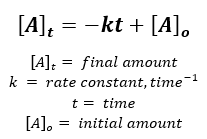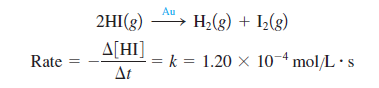# Problem: The decomposition of hydrogen iodide on finely divided gold at 150°C is zero order with respect to HI. The rate defined below is constant at 1.20 x 10-4 mol/L•s.a. If the initial HI concentration was 0.250 mol/L, calculate the concentration of HI at 25 minutes after the start of the reaction.

###### FREE Expert Solution

Recall the equation used for a zero-order reaction:Given:

k = 1.20x10-4 mol/(L·s)

[HI]o = 0.250 mol/L

Calculate amount of HI at 25 min:

85% (471 ratings)###### Problem Details

The decomposition of hydrogen iodide on finely divided gold at 150°C is zero order with respect to HI. The rate defined below is constant at 1.20 x 10-4 mol/L•s.a. If the initial HI concentration was 0.250 mol/L, calculate the concentration of HI at 25 minutes after the start of the reaction.